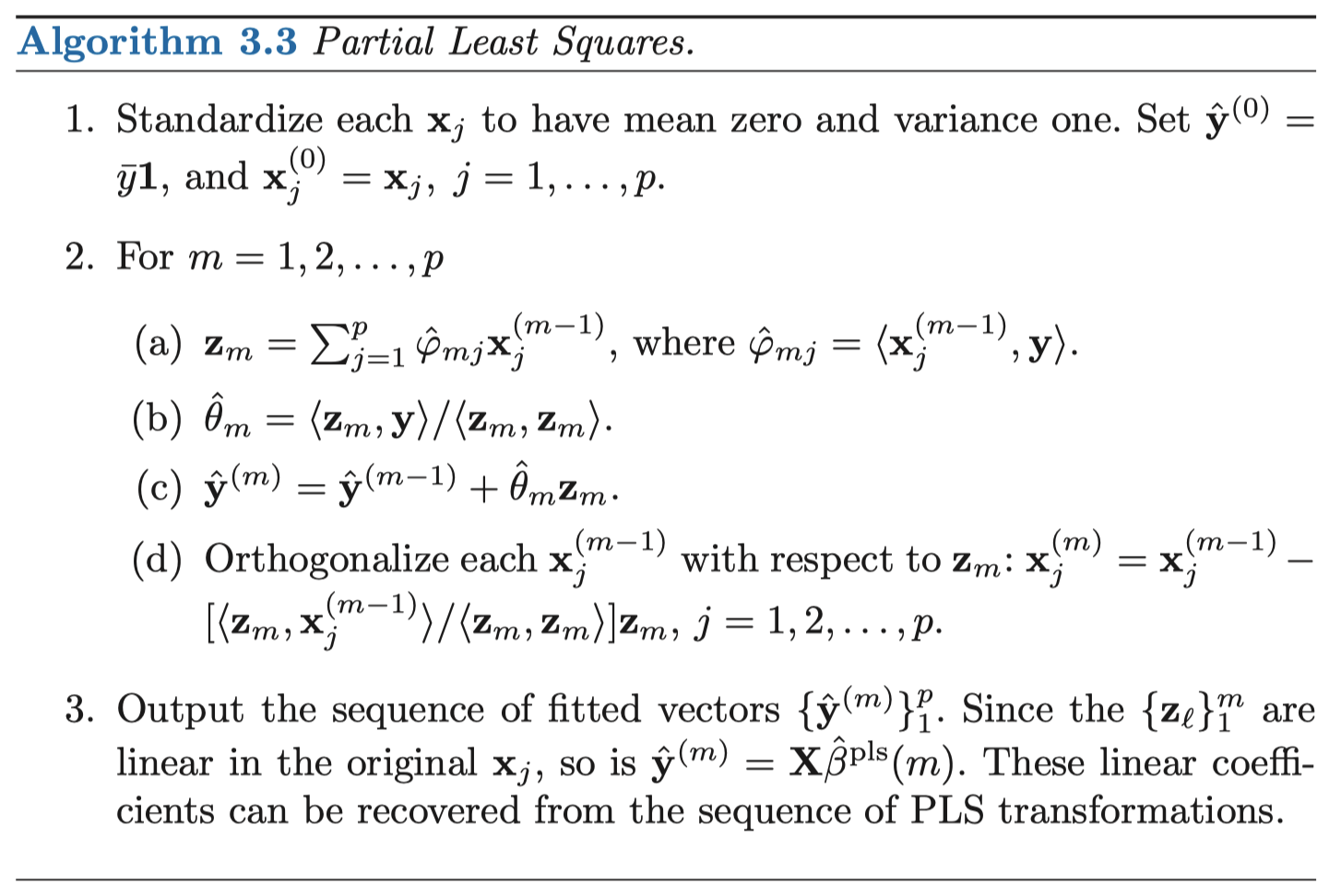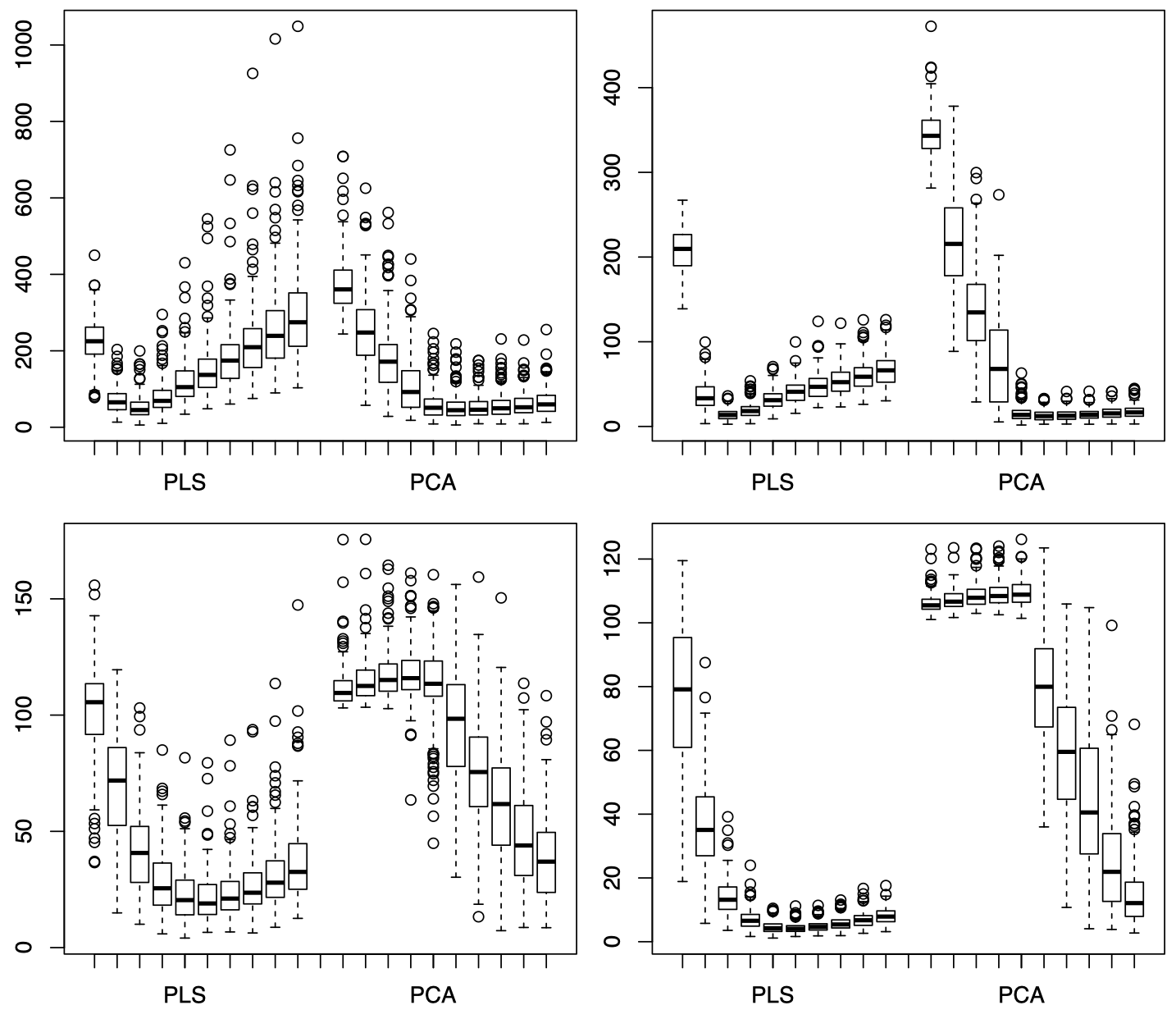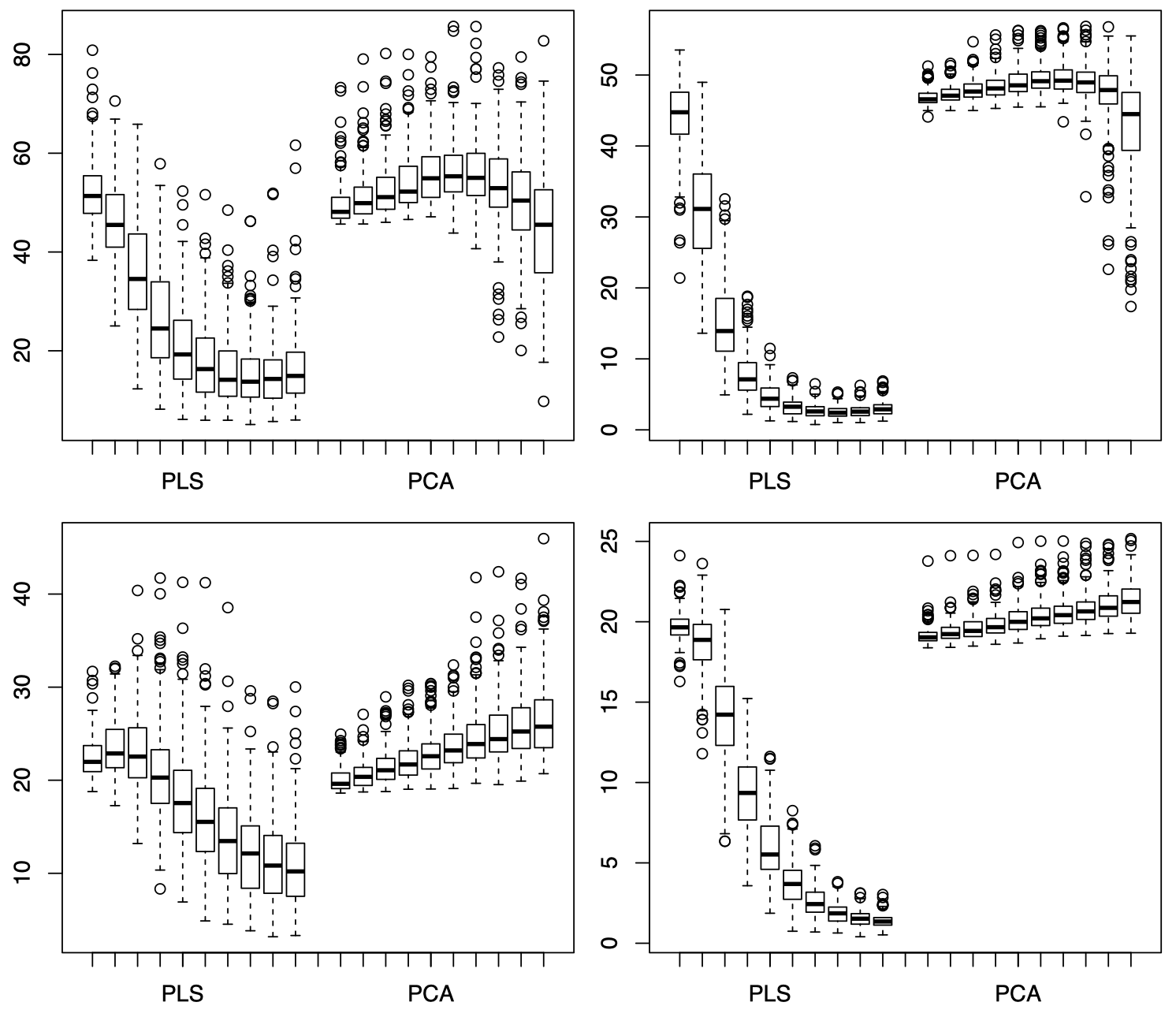# 数据科学多元统计学 06：主成分分析 (三)

## 墨尔本大学 MAST90138 课程笔记

Posted by YEY on September 8, 2020

# Lecture 06 主成分分析 (三)

• Hardle, W. and Simar, L (2015). Applied multivariate statistical analysis, 4th edition.
• Hastie, T. Tibshirani, R. and Friedman, J. (2009). The elements of statistical learning, 2nd edition

## 1. 主成分回归

\begin{align} Z &= \beta^{\mathrm T}X + \epsilon \\[2ex] &= \beta^{\mathrm T}\Gamma Y + \epsilon \\[2ex] &= \beta^{\mathrm T}\underbrace{\Gamma_{(1)}}_{p\times q} \underbrace{Y_{(1)}}_{q\times 1} + \beta^{\mathrm T} \underbrace{\Gamma_{(2)}}_{p\times (p-q)\;} \underbrace{Y_{(2)}}_{\;(p-q)\times 1} + \epsilon \end{align}

$Z = \beta_{\mathrm{PC}}^{\mathrm T} Y_{(1)} + \eta$

$\hat \beta^{\mathrm{PCR}}(q) = \hat \Gamma_{(1)} \hat \beta_{\mathrm{PC}}$

$\hat \beta_{\mathrm{LS}} = (\mathcal X^{\mathrm T}\mathcal X)^{-1}\mathcal X^{\mathrm T} \mathcal Z$

$\mathrm{Cov}(\hat \beta_{\mathrm{LS}}) - \mathrm{Cov}(\hat \beta^{\mathrm{PCR}}(q)) \ge 0$

(回忆一下，这意味着 “正定”)

## 2. 偏最小二乘法

$Z=m(X) + \epsilon\; , \quad m(x)= E(Z\mid X=x) = \beta^{\mathrm T}x$

$Y_j = \gamma_j^{\mathrm T}X \, , \;\; Y=\Gamma^{\mathrm T}X$

$m(x) = \beta^{\mathrm T}x \approx m_{\mathrm{PC}}(y_{(1)}) = \beta_{\mathrm{PC}}^{\mathrm T}y_{(1)}$

$T_1,T_2,\dots,T_p$

• 在 PCA 中，第一个成分 $Y_1$ 通过下式构造：

$Y_1 = \gamma_1^{\mathrm T} X$

其中，$\|\gamma_1\| = 1$，并且 $\mathrm{Var}(Y_1)$ 尽可能大。

• 在 PLS 中，第一个成分 $T_1$ 通过下式构造：

$T_1 = \phi_1^{\mathrm T} X$

其中，$\|\phi_1 \| = 1$，并且 $|\mathrm{Cov}(Z,T_1) |$ 尽可能大。

注意：$Z$ 和 $T_1$ 都是 $1$ 维的，而 $\phi_1$ 是一个 $p$ 维向量。

• 然后，对于 $k=2,\dots,p$，第 $k$ 个成分 $T_k$ 通过下式构造：

$T_k = \phi_k^{\mathrm T} X$

其中，$\|\phi_k \| = 1$，

$\phi_k^{\mathrm T} \Sigma \phi_j =0 \quad \text{for}\; j=1,\dots,k-1$

并且 $|\mathrm{Cov}(Z,T_k) |$ 尽可能大。

注意：$Z$ 和 $T_k$ 都是 $1$ 维的，而 $\phi_k$ 是一个 $p$ 维向量。

$X$ 在 $T_j$ 上的投影被构造为仍然保持 $X$ 的线性组合的形式，并且这些线性组合解释了 $Z$ 和 $X$ 之间的大部分线性关系。

$m(x) = E(Z\mid X=x) = \beta^{\mathrm T}x$

$t=(t_1,\dots,t_p)^{\mathrm T} =\Phi^{\mathrm T} x$

$T_{(1)} = (T_1,\dots,T_q)^{\mathrm T} \quad \text{and} \quad t_{(1)} = (t_1,\dots,t_q)^{\mathrm T}$

$m(x) = \beta^{\mathrm T}x \approx E(Z\mid T_{(1)}=t_{(1)}) \equiv m_{\mathrm{PLS}}(t_{(1)}) = \beta_{\mathrm{PLS}}^{\mathrm T} t_{(1)}$

$\beta_{\mathrm{PLS}}$ 的最小二乘估计量等于

$\hat \beta_{\mathrm{PLS}} =\mathop{\operatorname{arg\,min}}\limits_{b\in \mathbb R^q} \sum_{i=1}^{n}(Z_i - b^{\mathrm T} T_{(1),i})^2 = (\mathcal T_{(1)}^{\mathrm T}\mathcal T_{(1)})^{-1} \mathcal T_{(1)}^{\mathrm T} \mathcal Z$

$T_{(1),i} = (T_{i1},\dots,T_{iq})^{\mathrm T}$

$\mathcal T_{(1)} = \begin{pmatrix}T_{11} & \cdots & T_{1q} \\ \vdots & \ddots & \vdots \\ T_{n1} & \cdots & T_{nq}\end{pmatrix}$

$q=\mathop{\operatorname{arg\,min}}\limits_{k=1,\dots,p} CV(k)$

$CV(k) = \sum_{i=1}^{n} \{Z_i - \hat \beta_{\mathrm{PLS},k}^{-i} T_{(1),i}\}^2$## 3. 讨论：PCA vs. PLS

PCA 和 PLS 也可以用于非线性回归模型，我们同样可以将 $m(x) = E(Z\mid X=x)$ 近似为：

$m_{\mathrm{PLS}}(t_{(1)}) = E(Z\mid T_{(1)} = t_{(1)}) \quad \text{or} \quad m_{\mathrm{PC}}(y_{(1)}) = E(Z\mid Y_{(1)} = y_{(1)})$

### 例子：音素数据 (Phoneme data)

$X_i \in \mathbb R^{256}$ 代表由音素 “aa” (例如 “dark”) 和 “ao” (例如“water”) 录音构成的对数周期。

$Z_i = m(X_i) + \epsilon_i = \beta^{\mathrm T}X_i + \epsilon_i$

• 情况 (i)：信息包含在前 5 个 PC 中
• 情况 (ii)：信息包含在第 6 到第 10 个 PC 中
• 情况 (iii)：信息包含在第 11 到第 15 个 PC 中
• 情况 (iv)：信息包含在第 16 到第 20 个 PC 中

$\hat Z_{i}^{\mathrm{PLS}} = \hat m_{\mathrm{PLS}}(T_{(1),i}) \quad \text{or} \quad \hat Z_{i}^{\mathrm{PC}} = \hat m_{\mathrm{PC}}(Y_{(1),i})$

$\text{for} \; q=1,2,\dots,10$.

$PE = \dfrac{1}{N-n}\sum_{i=1}^{N-n}(Z_i - \hat Z_i)^2$本作品采用知识共享署名-非商业性使用-相同方式共享 4.0 国际许可协议进行许可。 欢迎转载，并请注明来自：YEY 的博客 同时保持文章内容的完整和以上声明信息！